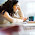## Monday, June 24, 2013

### Sound formal systems with sufficiently expressive languages are incomplete

In chapter 5, Peter Smith uses a counting argument to prove that sound axiomatized formal theories (AFTs) that can express a good amount of arithmetic are incomplete. In short: since their set of theorems is effectively enumerable but their set of true sentences is not, the two sets must be different. In a sound theory, the theorems are all true. Therefore, some truths must remained unproved in such theories.

Let's start with a definition. The language of an AFT of arithmetic is sufficiently expressive if and only if:
1. It can express quantification over numbers, and
2. It can express every effectively decidable two-place numerical relation.
Now, to the pivotal theorem of this chapter:
Theorem 1: The set of true sentences of a sufficiently expressive language of arithmetic is not effectively enumerable.
Proof sketch:
• According to the previous post, the set K is effectively enumerable. Therefore, there is an effectively computable function f from  to K that enumerates K.
• Now, consider the binary relation R(x,y) that is true if and only if f(x)=y. Then, n ∈ K if and only if there exists a number x such that R(x,n) holds.
• Since f is effectively computable, R is effectively decidable; so, by definition, a sufficiently expressive (interpreted) language L can express R and the existential quantifier. Let ∃x R(x,n) be a sentence of L that expresses "there exists a number x such that R(x,n) holds."
• In short: n ∈ K if and only if ∃x R(x,n) is true in L's interpretation.
• That is: n ∉ K if and only if ¬ ∃x R(x,n) is true in L's interpretation.
• If the set of true sentences of L were effectively enumerable, then we could go through and, for each formula of the form ¬ ∃x R(x,n), we could output the value of n (while skipping all of the other sentences). But this algorithm would constitute an effective enumeration of K. Since we proved that K is not effectively enumerable, the set of true sentences of L is not effectively enumerable.

The main result of this post follows easily from Theorem 1.

Let's call S1 the set of theorems of an AFT built on top of a sufficiently expressive language L. We know that S1 is effectively enumerable. But, according to theorem 1, the set S2 of the true sentences of L is not effectively enumerable. From this simple counting argument, we can infer that S1 ≠ S2. So, either some theorems are not true or some true sentences are not theorems.

If we assume that the AFT is sound, then it must be the case that S1 ⊂ S2. Therefore, there must exist at least one true sentence φ in L that is not a theorem. In addition, since φ is true, ¬ φ is false and thus cannot be a theorem either. In conclusion, neither φ nor ¬ φ is a theorem. This is a proof of:
Theorem 2: Sound AFTs of arithmetic whose language is sufficiently expressive are negation-incomplete.

1.2.